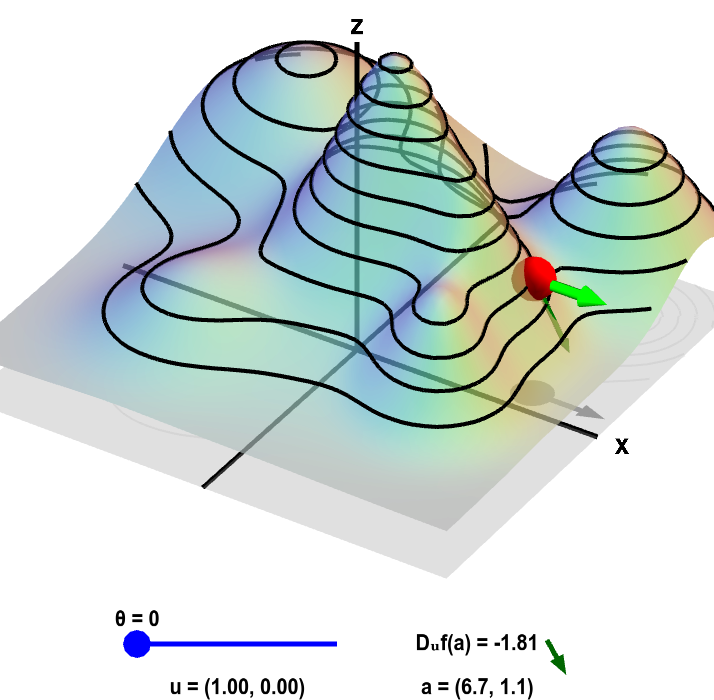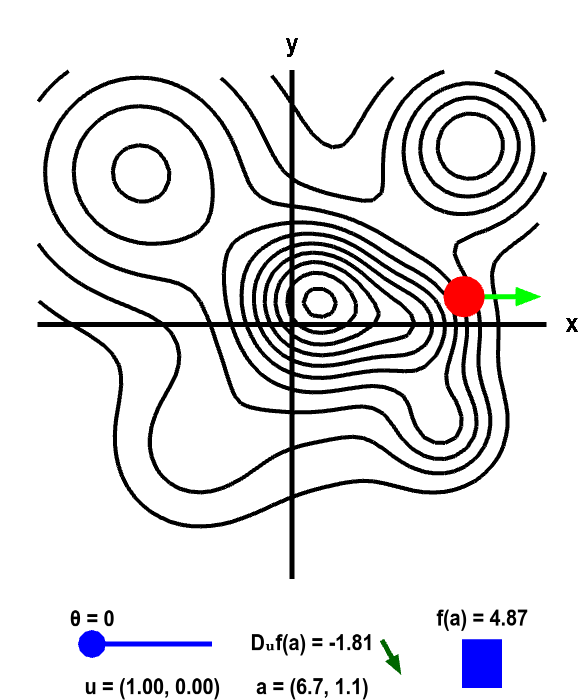# Math Insight

### Applet: Directional derivative on a mountainThe height of a mountain range described by a function $f(x,y)$ is shown as surface plot in three-dimensions (left) and a two-dimensional level curve plot (right). In each panel, a red point can be moved by the mouse to change where the directional derivative is evaluated. The directional derivative is computed in the direction of the two-dimensional vector $\vc{u}$. This direction is illustrated by the light green vectors, and the value of the direction $\vc{u}$ is shown in the lower left of each panel. The direction of $\vc{u}$ is determined by the angle $\theta$ it makes with straight east (positive $x$ direction). The angle $\theta$, and hence $\vc{u}$, can be changed using the slider. The two-dimensional point $\vc{a}$ where the directional derivative is computed is illustrated by the shadow of the red point on the $xy$-plane below the surface plot and by the red point itself on the level curve plot. The value of the directional derivative $D_{\vc{u}}f(\vc{a})$ is shown at the bottom of the panel, along with the value of $\vc{a}$ itself. The value of $D_{\vc{u}}f(\vc{a})$ is the slope of the dark green vector to its right. This dark green vector is also shown emanating from the red point on the surface plot, where it is tangent to the surface, indicating that this slope is indeed the slope of the surface in the direction given by $\vc{u}$. The height of the surface $f(\vc{a})$ is illustrated by the bar in the lower right of the second panel.